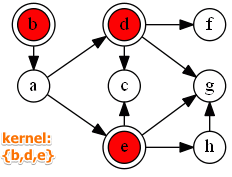# ML Wiki

## Graph Kernel

A kernel of a graph $K \subset V$ is ($V$ - all nodes of a graph)

• $\forall a \in K, \not \exists b: a \to b$
no alternative $a$ inside the kernel $K$ is better than any other alternative $b$ inside $K$
• $\forall c \not \in K, \exists a \in K: a \to c$
each alternative $c$ outside of the kernel $K$ is worse than at least one alternative $a$ inside $K$

For the example above:

• $K = \{b, d, e\}$
•Remarks: